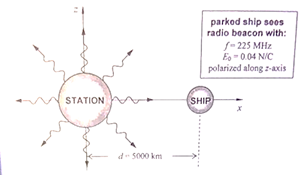# Problem: Calculate the total average power of the station's radio transmitter. a. &lt;P&gt; = 330 MW b. &lt;P&gt; = 485 MW c. &lt;P&gt; = 667 MW d. &lt;P&gt; = 802 MW e. &lt;P&gt; = 950 MW

###### FREE Expert Solution
86% (456 ratings)
###### Problem Details

Calculate the total average power of the station's radio transmitter.

a. <P> = 330 MW

b. <P> = 485 MW

c. <P> = 667 MW

d. <P> = 802 MW

e. <P> = 950 MW